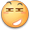# 2019-4-19-div2 cf ，又是一次显示我的愚蠢的比赛

## A. Maxim and BiologyToday in the scientific lyceum of the Kingdom of Kremland, there was a biology lesson. The topic of the lesson was the genomes. Let's call the genome the string "ACTG".

Maxim was very boring to sit in class, so the teacher came up with a task for him: on a given string s consisting of uppercase letters and length of at least 4, you need to find the minimum number of operations that you need to apply, so that the genome appears in it as a substring. For one operation, you can replace any letter in the string s with the next or previous in the alphabet. For example, for the letter "D" the previous one will be "C", and the next — "E". In this problem, we assume that for the letter "A", the previous one will be the letter "Z", and the next one will be "B", and for the letter "Z", the previous one is the letter "Y", and the next one is the letter "A".

Help Maxim solve the problem that the teacher gave him.

A string a is a substring of a string b if can be obtained from b by deletion of several (possibly, zero or all) characters from the beginning and several (possibly, zero or all) characters from the end.

A题是一很简单的暴力然而我就是在预处理的时候处理不出来我想要的结果

## B. Dima and a Bad XORStudent Dima from Kremland has a matrix a of size n×m filled with non-negative integers.

He wants to select exactly one integer from each row of the matrix so that the bitwise exclusive OR of the selected integers is strictly greater than zero. Help him!

Formally, he wants to choose an integers sequence c1,c2,,cn (1cjm) so that the inequality a(1,c1)a(2,c2)a(n,cn)>0holds, where a(i,j) is the matrix element from the i-th row and the j-th column.

Here xy denotes the bitwise XOR operation of integers xx and yy.

b题的话我我明显发现我的智商偏低了

## C. Problem for NazarNazar, a student of the scientific lyceum of the Kingdom of Kremland, is known for his outstanding mathematical abilities. Today a math teacher gave him a very difficult task.

Consider two infinite sets of numbers. The first set consists of odd positive numbers (1,3,5,7,), and the second set consists of even positive numbers (2,4,6,8,). At the first stage, the teacher writes the first number on the endless blackboard from the first set, in the second stage — the first two numbers from the second set, on the third stage — the next four numbers from the first set, on the fourth — the next eight numbers from the second set and so on. In other words, at each stage, starting from the second, he writes out two times more numbers than at the previous one, and also changes the set from which these numbers are written out to another.

The ten first written numbers: 1,2,4,3,5,7,9,6,8,10. Let's number the numbers written, starting with one.

The task is to find the sum of numbers with numbers from ll to r for given integers ll and r. The answer may be big, so you need to find the remainder of the division by 1000000007 (109+7).

Nazar thought about this problem for a long time, but didn't come up with a solution. Help him solve this problem.

## D. Stas and the Queue at the BuffetDuring a break in the buffet of the scientific lyceum of the Kingdom of Kremland, there was formed a queue of n high school students numbered from 1 to n. Initially, each student i is on position i. Each student i is characterized by two numbers — ai and biDissatisfaction of the person i equals the product of ai by the number of people standing to the left of his position, add the product bi by the number of people standing to the right of his position. Formally, the dissatisfaction of the student i, which is on the position j, equals ai⋅(j−1)+bi⋅(n−j).

The director entrusted Stas with the task: rearrange the people in the queue so that minimize the total dissatisfaction.

Although Stas is able to solve such problems, this was not given to him. He turned for help to you.

d题的话因为b题消耗时间太多，在加上没有读懂题，所以最后16分钟开的D题，虽说最后还是没有写出来吧d题的思路也很简单定义一个含有三个元素的结构体，a,b,x。a是储存在a数里相应的值，b是储存在b数组里相应的值，令  x = a - b;

1.自己真菜，

2.死磕在了b题上

3.写代码的速度还是有点慢

4.写题的第一想法是暴力，应该在多想想，比如b题如果能想出来第一列异或和为0只需要在i(1~n)行中找一个能替换的输出就好，而不是下意识的直接想起来一个n^m的暴力

5.还没想好，等我补完剩下的三道题在来看看还有没有补充的吧

5.还没想好,不过剩下的那两题超出了我的实力范围之内,果断跳过。